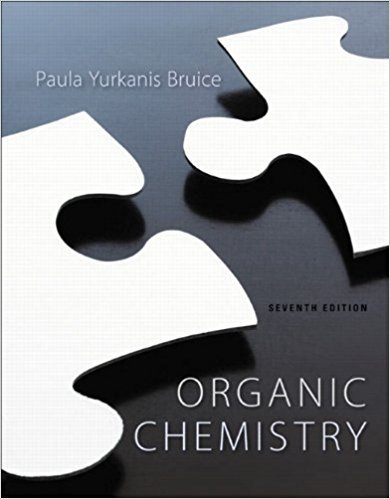×
×

# The reaction of chloromethane with hydroxide ion at 30 oCISBN: 9781269406772 80

## Solution for problem 68P Chapter 9

Organic Chemistry | 7th Edition

• Textbook Solutions
• 2901 Step-by-step solutions solved by professors and subject experts
• Get 24/7 help from StudySoup virtual teaching assistantsOrganic Chemistry | 7th Edition

4 5 1 355 Reviews
28
5
Problem 68P

The reaction of chloromethane with hydroxide ion at 30 oC has a ΔGo value of -21.7 kcal/mol. What is the equilibrium constant for the reaction?

Step-by-Step Solution:

Solution 68P

Step 1 of 5</p>

For a reaction of chloromethane with hydroxide ion:The above reaction is belongs toreaction. So, the rate is depends upon both nucleophile and chloromethane.Here ‘’is known as the rate constant for the reaction. The reaction is said to second order overall. That is first order with respect toand first order with respect to.

Step 2 of 5</p>

The change in free energy in going from starting materials to products,is related to the equilibrium constant, for the change by the relation,Here,is called the free energy of activation for a reaction.’ is the universal gas constant.’ is the absolute temperature.

Step 3 of 5</p>

That is larger the decrease in free energy (minus) on going from starting materials to products, the larger the value of, and the equilibrium lies in favour of the products. The position of minimum free energy thus corresponds to the attainment of equilibrium by starting materials or products. In a reaction for which there is no free energy change, which corresponds toconversion of starting materials into products. Increasing positive values ofrapidly decreases value of, corresponding to extremely little conversion into products, while increasing negative values ofcorresponds to rapid increase ofvalues.

Step 4 of 5

Step 5 of 5

##### ISBN: 9781269406772

Unlock Textbook Solution